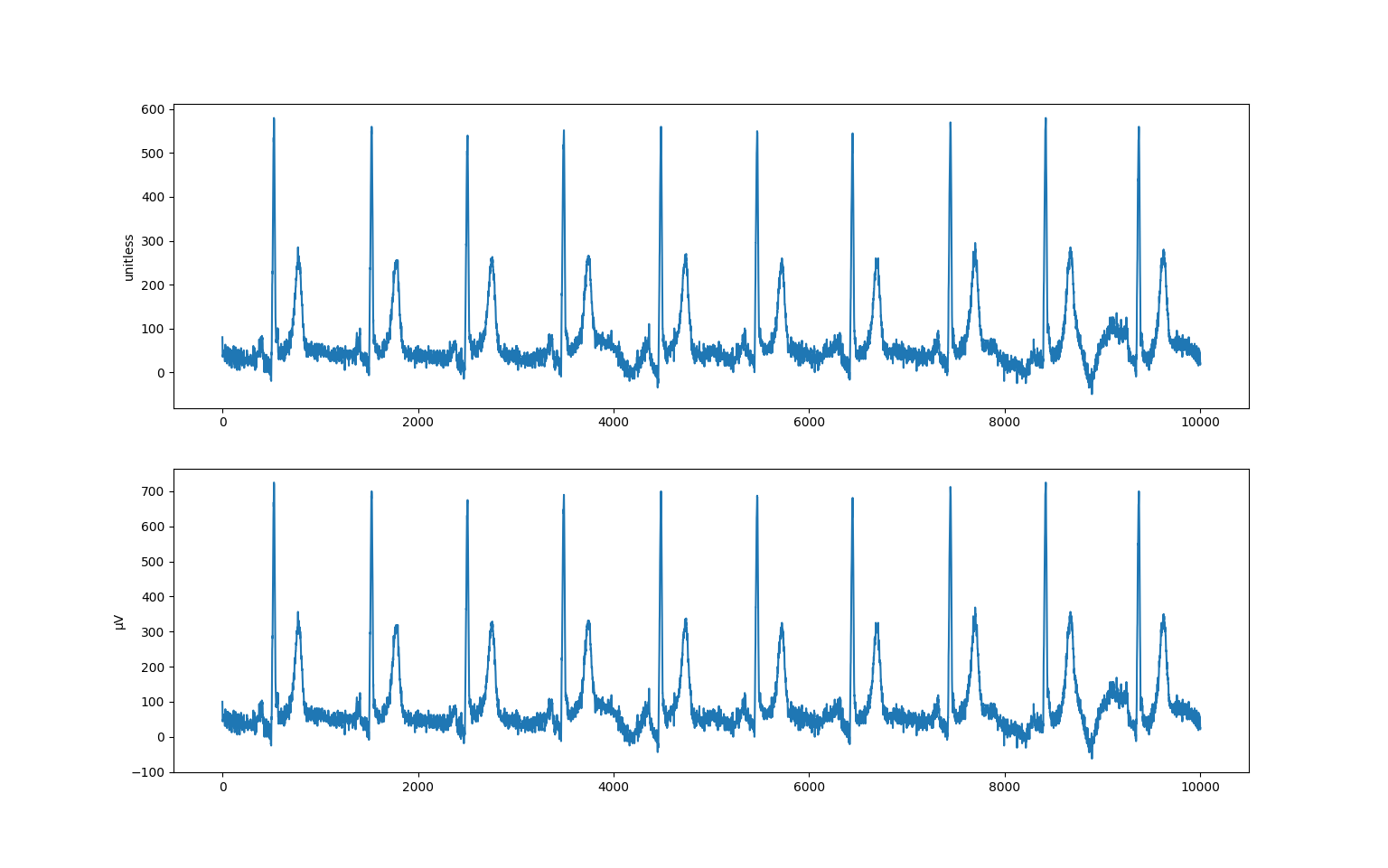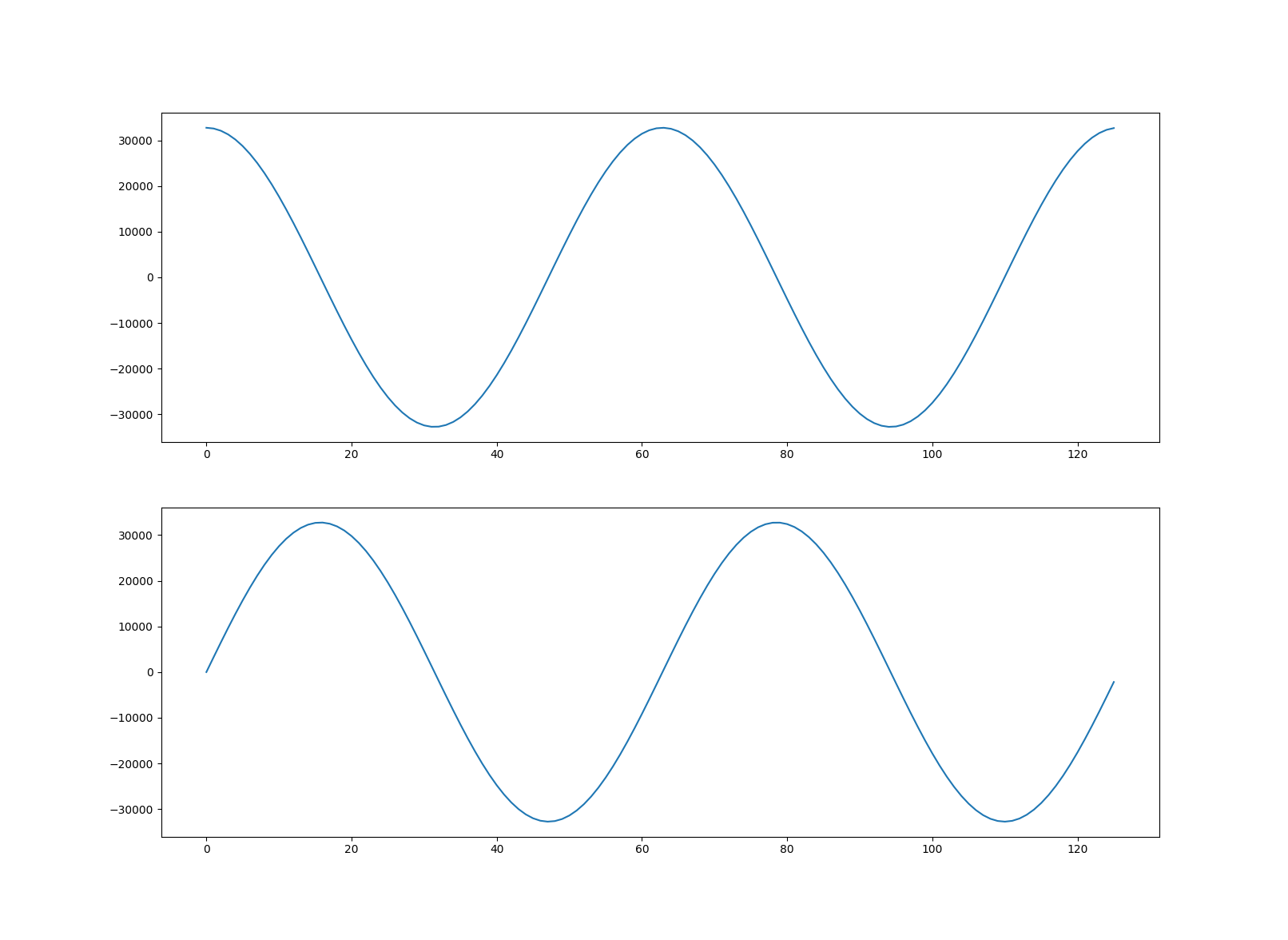# Waveforms¶

This tutorial is about understanding waveforms in DICOM datasets and covers:

• An introduction to DICOM waveforms

• Decoding and displaying Waveform Data

• Encoding Waveform Data

It’s assumed that you’re already familiar with the dataset basics.

Prerequisites

```python -m pip install -U pydicom>=2.1 numpy matplotlib
```
```conda install numpy matplotlib
conda install -c conda-forge pydicom>=2.1
```

References

## Waveforms in DICOM¶

There are a number of DICOM Information Object Definitions (IODs) that contain waveforms, such as 12-Lead ECG, Respiratory Waveform and Real-Time Audio Waveform. Every waveform IOD uses the Waveform Module to represent one or more multi-channel time-based digitized waveforms, sampled at constant time intervals.

The waveforms within a dataset are contained in the items of the (5400,0100) Waveform Sequence element:

```>>> from pydicom import dcmread
>>> from pydicom.data import get_testdata_file
>>> fpath = get_testdata_file("waveform_ecg.dcm")
>>> ds.SOPClassUID.name
>>> waveforms = ds.WaveformSequence
>>> len(waveforms)
2
```

Each item in the sequence is a multiplex group, which is a group of related waveforms that are synchronised at common sampling frequency.

```>>> multiplex = waveforms
>>> multiplex.MultiplexGroupLabel
'RHYTHM'
>>> multiplex.SamplingFrequency  # in Hz
"1000.0"
>>> multiplex.NumberOfWaveformChannels
12
>>> multiplex.NumberOfWaveformSamples
10000
```

So the first multiplex group has 12 channels, each with 10,000 samples. Since the sampling frequency is 1 kHz, this represents 10 seconds of data. The defining information for each channel is available in the (5400,0200) Channel Definition Sequence:

```>>> for ii, channel in enumerate(multiplex.ChannelDefinitionSequence):
...     source = channel.ChannelSourceSequence.CodeMeaning
...     units = 'unitless'
...     if 'ChannelSensitivity' in channel:  # Type 1C, may be absent
...         units = channel.ChannelSensitivityUnitsSequence.CodeMeaning
...     print(f"Channel {ii + 1}: {source} ({units})")
...
Channel 1: Lead I (Einthoven) (microvolt)
```

## Decoding Waveform Data¶

The combined sample data for each multiplex is stored in the corresponding (5400,1010) Waveform Data element:

```>>> multiplex.WaveformBitsAllocated
16
>>> multiplex.WaveformSampleInterpretation
'SS'
>>> len(multiplex.WaveformData)
240000
```

If Waveform Bits Allocated is `16` and Waveform Sample Interpretation is `'SS'` then the data for this multiplex consists of signed 16-bit samples. Waveform data is encoded with the channels interleaved, so for our case the data is ordered as:

```(Ch 1, Sample 1), (Ch 2, Sample 1), ..., (Ch 12, Sample 1),
(Ch 1, Sample 2), (Ch 2, Sample 2), ..., (Ch 12, Sample 2),
...,
(Ch 1, Sample 10,000), (Ch 2, Sample 10,000), ..., (Ch 12, Sample 10,000)
```

To decode the raw multiplex waveform data to a numpy `ndarray` you can use the `multiplex_array()` function. The following decodes and returns the raw data from the multiplex at index `0` within the Waveform Sequence:

```>>> from pydicom.waveforms import multiplex_array
>>> raw = multiplex_array(ds, 0, as_raw=True)
>>> raw[0, 0]
80
```

If (003A,0210) Channel Sensitivity is present within the multiplex’s Channel Definition Sequence then the raw sample data needs to be corrected before it’s in the quantity it represents. This correction is given by (sample + Channel Baseline) x Channel Sensitivity x Channel Sensitivity Correction Factor and will be applied when as_raw is `False` or when using the `Dataset.waveform_array()` function:

```>>> arr = ds.waveform_array(0)
>>> arr[0, 0]
>>> 100.0
>>> import matplotlib.pyplot as plt
>>> fig, (ax1, ax2) = plt.subplots(2)
>>> ax1.plot(raw[:, 0])
>>> ax1.set_ylabel("unitless")
>>> ax2.plot(arr[:, 0])
>>> ax2.set_ylabel("μV")
>>> plt.show()
```When processing large amounts of waveform data it might be more efficient to use the `generate_multiplex()` function instead. It yields an `ndarray` for each multiplex group within the Waveform Sequence:

```>>> from pydicom.waveforms import generate_multiplex
>>> for arr in generate_multiplex(ds, as_raw=False):
...     print(arr.shape)
...
(10000, 12)
(1200, 12)
```

## Encoding Waveform Data¶

Having seen how to decode and view a waveform then next step is creating our own multiplex group. The new group will contain two channels representing cosine and sine curves. We’ve chosen to represent our waveforms using signed 16-bit integers, but you can use signed or unsigned 8, 16, 32 or 64-bit integers depending on the requirements of the IOD.

First we create two `ndarrays` with our waveform data:

```>>> import numpy as np
>>> x = np.arange(0, 4 * np.pi, 0.1)
>>> ch1 = (np.cos(x) * (2**15 - 1)).astype('int16')
>>> ch2 = (np.sin(x) * (2**15 - 1)).astype('int16')
```

Next we create the new multiplex group that will contain the waveforms:

```>>> from pydicom.dataset import Dataset
>>> new = Dataset()
>>> new.WaveformOriginality = "ORIGINAL"
>>> new.NumberOfWaveformChannels = 2
>>> new.NumberOfWaveformSamples = len(x)
>>> new.SamplingFrequency = 1000.0
```

To find out which elements we need to add to our new multiplex, we check the Waveform Module in Part 3 of the DICOM Standard. Type 1 elements must be present and not empty, Type 1C are conditionally required, Type 2 elements must be present but may be empty, and Type 3 elements are optional.

Set our channel definitions, one for each channel (note that we have opted not to include a Channel Sensitivity, so our data will be unit-less). If you were to do this for real you would obviously use an official coding scheme.

```>>> new.ChannelDefinitionSequence = [Dataset(), Dataset()]
>>> chdef_seq = new.ChannelDefinitionSequence
>>> for chdef, curve_type in zip(chdef_seq, ["cosine", "sine"]):
...     chdef.ChannelSampleSkew = "0"
...     chdef.WaveformBitsStored = 16
...     chdef.ChannelSourceSequence = [Dataset()]
...     source = chdef.ChannelSourceSequence
...     source.CodeValue = "1.0"
...     source.CodingSchemeDesignator = "PYDICOM"
...     source.CodingSchemeVersion = "1.0"
...     source.CodeMeaning = curve_type
```

Interleave the waveform samples, convert to bytes and set the Waveform Data. Since the dataset’s transfer syntax is little endian, if you’re working on a big endian system you’ll need to perform the necessary conversion. You can determine the endianness of your system with ```import sys; print(sys.byteorder)```.

We also set our corresponding Waveform Bits Allocated and Waveform Sample Interpretation element values to match our data representation type:

```>>> arr = np.stack((ch1, ch2), axis=1)
>>> arr.shape
(126, 2)
>>> new.WaveformData = arr.tobytes()
>>> new.WaveformBitsAllocated = 16
>>> new.WaveformSampleInterpretation = 'SS'
```

And finally add the new multiplex group to our example dataset and save:

```>>> ds.WaveformSequence.append(new)
>>> ds.save_as("my_waveform.dcm")
```

We should now be able to plot our new waveforms:

```>>> ds = dcmread("my_waveform.dcm")
>>> arr = ds.waveform_array(2)
>>> fig, (ax1, ax2) = plt.subplots(2)
>>> ax1.plot(arr[:, 0])
>>> ax2.plot(arr[:, 1])
>>> plt.show()
```